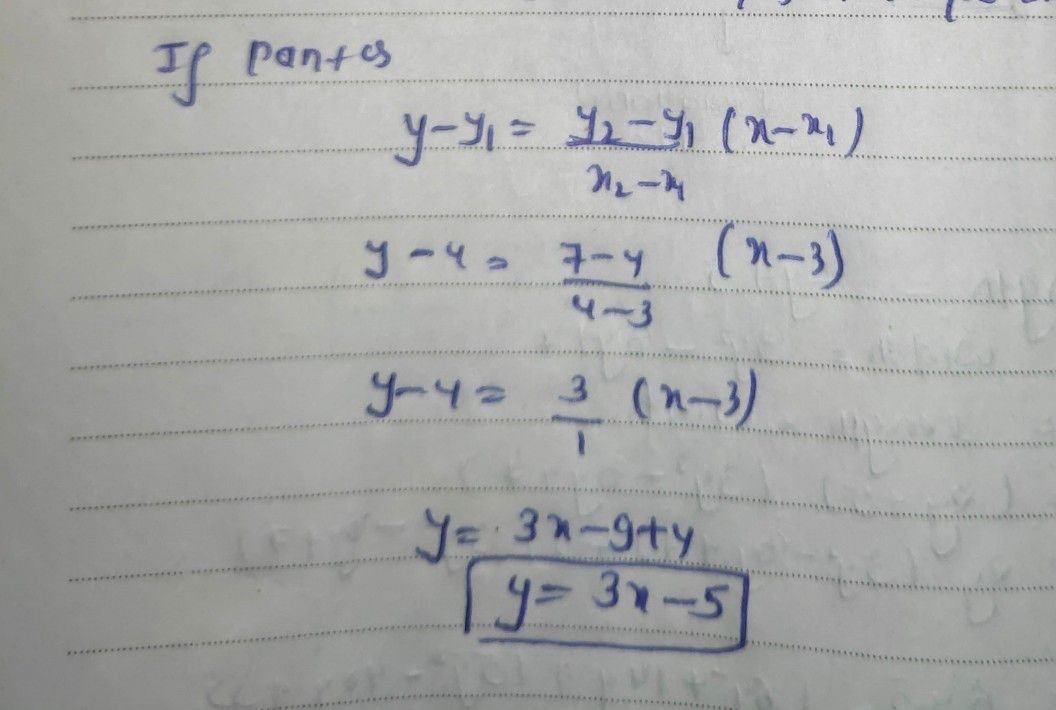Symbol
ProblemExercise 1. Find the equation of the line of the form $y=mx+b$ that passes through the following $De1rs$ of points. $0se$ separate sheet $for$ $y04$ answers.) $1.\right)$ $\left(3,4\right)and\left(4$ $7\right)$ $2\right)$ $\left(8$ $4\right)and\left(6$ $10\right)$ $3.\right)$ $\left(3,-1\right)$ $4\right)$ $\left(-8$ $-1\right)and\left(7,-5\right)$ $5\right)and\left(-9,11\right)$